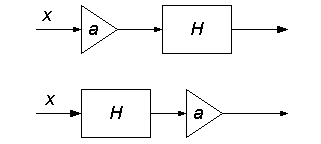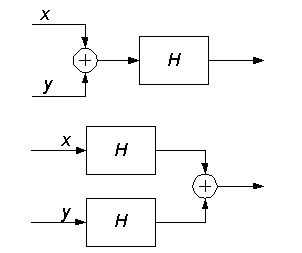EECS20N: Signals and Systems

# Linearity

Consider the set of signals whose range is Reals or Complex. Such signals are called real-valued functions or complex-valued functions. Since real-valued functions are a subset of complex-valued functions, we only need to talk about complex-valued functions. Suppose x is a complex-valued function and a is a complex constant. Then we can define a new complex-valued function ax such that for t in the domain of x,

(ax)(t) = a(x(t)).

In other words, the new function, which we call ax, is simply scaled by the constant a. For example, suppose x is the red signal in the applet below. You can vary a using the slider.

If you were able to run applets, you would have a Delay demo here.

Similarly, given two complex-valued functions x and y, we can define a new function (x + y) such that for t in the domain of x,

(x + y)(t) = x(t) + y(t).

Consider the set of all systems that map complex-valued functions into complex-valued functions. Such systems are called complex systems. Suppose that H is a complex system. H is said to be linear if for all aComplex and for all x and y that are complex-valued functions,

H(ax) = aH(x)

and

H(x + y) = H(x) + H(y).

The first of these says that if you scale the input, the output is scaled. The second one says that if the input is described as the sum of two component signals, then the output can be described as the sum of two signals that would result from the components alone. In pictures, the first property says that the following two systems are equivalent if H is linear:Here, the triangle represents the scaling operation. The second property says that the following two systems are equivalent:Linearity is a mathematical fiction. No man-made electronic system is linear in the strict sense. A system is designed to work with a range of input signals, and arbitrary scaling of the input does not translate into arbitrary scaling of the output. If you provide an input to your audio amplifier that is higher voltage than it is designed for, then it is not likely to just produce louder sounds. It is more likely to fry, perhaps producing one last glorious burst of sound before dying.

However, as a mathematical fiction, linearity is extremely convenient. It says that we can decompose the inputs to a system and study the effect of the system on the individual components.